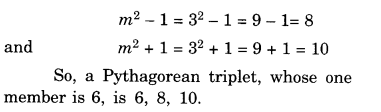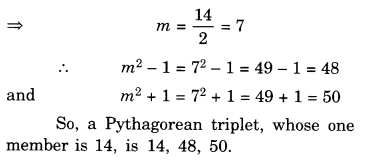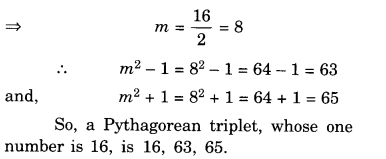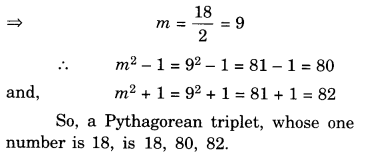NCERT Solutions for Class 8 Maths Chapter 6 Squares and Square Roots Ex 6.2 are part of NCERT Solutions for Class 8 Maths. Here we have given NCERT Solutions for Class 8 Maths Chapter 6 Squares and Square Roots Ex 6.2.

 Board CBSE Textbook NCERT Class Class 8 Subject Maths Chapter Chapter 6 Chapter Name Squares and Square Roots Exercise Ex 6.2 Number of Questions Solved 2 Category NCERT Solutions

## NCERT Solutions for Class 8 Maths Chapter 6 Squares and Square Roots Ex 6.2

Question 1.
Find the square of the following numbers:
(i) 32
(ii) 35
(iii) 86
(iv) 93
(v) 71
(vi) 46
Solution.
(i) 32
32 = 30 + 2
Therefore, $${ 32 }^{ 2 }$$ = $${ \left( 30+2 \right) }^{ 2 }$$
= 30 (30 + 2) + 2 (30 + 2)
= 900 + 60 + 60 + 4
= 1024

(ii) 35
35 = 30 + 5
Therefore, $${ 35 }^{ 2 }$$ = $${ \left( 30+5 \right) }^{ 2 }$$
= 30 (30 + 5) + 5 (30 + 5)
= 900 + 150 + 150 + 25
= 1225

(iii) 86
86 = 80 + 6
Therefore, $${ 86 }^{ 2 }$$= $${ \left( 80+6 \right) }^{ 2 }$$
= 80 (80 + 6) + 6 (80 + 6)
= 6400 + 480 + 480 + 36
= 7396

(iv) 93
93 = 90 + 3
Therefore, $${ 90 }^{ 2 }$$= $${ \left( 90+3\right) }^{ 2 }$$
= 90 (90 + 3) + 3 (90 + 3)
= 8100 + 270 + 270 + 9
= 8649

(v) 71
71 = 70 + 1
Therefore, $${ 70 }^{ 2 }$$= $${ \left( 70+1\right) }^{ 2 }$$
= 70 (70 + 1) + 1 (70 + 1)
= 4900 + 70 + 70 + 1
= 5041

(vi) 46
46 = 40 + 6
Therefore, $${ 40 }^{ 2 }$$= $${ \left( 40+6\right) }^{ 2 }$$
= 40 (40 + 6) + 6 (40 + 6)
= 1600 + 240 + 240 + 36
= 2116

Question 2.
Write a Pythagorean triplet whose one number is
(i) 6
(ii) 14
(iii) 16
(iv) 18
Solution.
(i) 6
Let 2m = 6
⇒ $$m=\frac { 6 }{ 2 } =3$$(ii) 14
Let 2m = 14(iii) 16
Let 2m = 16(iv) 18
Let 2m = 18We hope the NCERT Solutions for Class 8 Maths Chapter 6 Squares and Square Roots Ex 6.2 are part help you. If you have any query regarding NCERT Solutions for Class 8 Maths Chapter 6 Squares and Square Roots Ex 6.2 are part, drop a comment below and we will get back to you at the earliest.